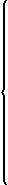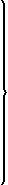# COMPUTE

#### Structured Mode Syntax[`ROUNDED`] {operand1 [:]= } ...{operand1 `:=` } ...#### Reporting Mode Syntax[`ROUNDED`]{operand1 [:]= }This document covers the following topics:

For an explanation of the symbols used in the syntax diagram, see Syntax Symbols.

Belongs to Function Group: Arithmetic and Data Movement Operations

## Function

The `COMPUTE` statement is used to perform an arithmetic or assignment operation.

A `COMPUTE` statement with multiple target operands (`operand1`) is identical to the corresponding individual `COMPUTE` statements if the source operand (`operand2`) is not an arithmetic expression.

`#TARGET1 := #TARGET2 := #SOURCE`

is identical to

```#TARGET1 := #SOURCE
#TARGET2 := #SOURCE```

Example:

```DEFINE DATA LOCAL
1 #ARRAY(I4/1:3) INIT <3,0,9>
1 #INDEX(I4)
1 #RESULT(I4)
END-DEFINE
*
#INDEX := 1
*
#INDEX := 		    /* #INDEX is 3
#RESULT := 		   /* #RESULT is 9
#ARRAY(#INDEX)
*
#INDEX := 2
*
#INDEX  := 		   /* #INDEX is 0
#ARRAY(3) := 	 	/* returns runtime error NAT1316
#ARRAY(#INDEX)
END```

If the source operand is an arithmetic expression, the expression is evaluated and its result is stored in a temporary variable. Then the temporary variable is assigned to the target operands.

```#TARGET1 := #TARGET2 := #SOURCE1 + 1
is identical to
#TEMP := #SOURCE1 + 1
#TARGET1 := #TEMP
#TARGET2 := #TEMP```

Example:

```DEFINE DATA LOCAL
1 #ARRAY(I4/1:3) INIT <2, 0, 9>
1 #INDEX(I4)
1 #RESULT(I4)
END-DEFINE
*
#INDEX := 1
*
#INDEX := 		/* #INDEX is 3
#RESULT := 		/* #RESULT is 3
#ARRAY(#INDEX) + 1
*
#INDEX := 2
*
#INDEX  :=		/* #INDEX is 0
#ARRAY(3) := 		/* returns run time error NAT1316
#ARRAY(#INDEX)
END```

For further information, see Rules for Arithmetic Assignment in the Programming Guide and particularly the following sections:

• Data Transfer (for information on data transfer compatibility and the rules for data transfer)

## Syntax Description

Operand Definition Table:

Operand Possible Structure Possible Formats Referencing Permitted Dynamic Definition
`operand1`   S A   M   A U N P I F B D T L C G O yes yes
`operand2` C S A   N E A U N P I F B D T L C G O yes no
`operand3` C S             N P I   B*             yes no
`operand4` C S             N P I   B*             yes no

* If `operand3` or `operand4` is a binary variable, it may be used only with a length of less than or equal to 4.

Syntax Element Description:

Syntax Element Description
``` COMPUTE | ASSIGN [:]=```
Usage of Keywords:

This statement may be issued in short form by omitting the statement keyword `COMPUTE` (or `ASSIGN`).

In structured mode, when the statement keyword `COMPUTE` (or `ASSIGN`) is omitted, the equal sign (=) must be preceded by a colon (:).

However, when the `ROUNDED` option is used, the statement keyword `COMPUTE` (or `ASSIGN`) must be specified.

`ROUNDED`
ROUNDED Option:

If you specify the keyword `ROUNDED`, the value will be rounded before it is assigned to `operand1`.

For information on rounding, see Rules for Arithmetic Assignments, Field Truncation and Field Rounding in the Programming Guide.

`operand1`
Result Field:

`operand1` will contain the result of the arithmetic/assignment operation.

For the precision of the result, see Precision of Results of Arithmetic Operations in the Programming Guide.

If `operand1` is a database field, the field in the database is not updated.

If `operand1` is a dynamic variable, it is filled with exactly the data and length of `operand2` or the length of the result of the arithmetic-operation, including trailing blanks. The current length of a dynamic variable can be obtained by using the system variable `*LENGTH`.

For general information on dynamic variables, see Using Dynamic and Large Variables.

`arithmetic-expression`
Arithmetic Expression:

An arithmetic expression consists of one or more constants, database fields, and user-defined variables.

Natural mathematical functions (described in the System Functions documentation) may also be used as arithmetic operands.

Operands used in an arithmetic expression must be defined with format N, P, I, F, D, or T.

As for the formats of the operands, see also Performance Considerations for Mixed Formats in the Programming Guide.

The following connecting operators may be used:

Operator: Symbol:
Parentheses `( )`
Exponentiation `**`
Multiplication `*`
Division `/`
Addition `+`
Subtraction `-`

Each operator should be preceded and followed by at least one blank so as to avoid any conflict with a variable name that contains any of the above characters.

The processing order of arithmetic operations is:

1. Parentheses

2. Exponentiation

3. Multiplication/division (left to right as detected)

4. Addition/subtraction (left to right as detected)

`operand2`
Source Field:

`operand2` is the source field. If `operand1` is of format C, `operand2` may also be specified as an attribute constant.

See User-Defined Constants in the Programming Guide.

``` SUBSTRING``` (operand2,operand3,operand4)
SUBSTRING Option:

Without the substring option, the whole content of `operand2` is moved.

If `operand1` and `operand2` are of alphanumeric, Unicode or binary format, you may use the `SUBSTRING` option to assign a certain part of `operand2` to `operand1`.

After the field name (`operand2`) in the `SUBSTRING` clause, you specify the starting position (`operand3`) and then the length (`operand4`) of the field portion to be moved.

For example, to assign the 3rd to 6th position of field `#B` to field `#A`, you would specify:

`#A := SUBSTRING(#B,3,4)`

If you omit `operand3`, the starting position is assumed to be `1`. If you omit `operand4`, the length is assumed to range from the starting position to the end of the field.

Note:
`ASSIGN` with the `SUBSTRING` option is a byte-by-byte assignment (that is, the rules described under Rules for Arithmetic Assignment in the Programming Guide do not apply).

## Result Precision of a Division

The precision (number of decimal positions) of the result of a division in a `COMPUTE` statement is determined by the precision of either the first operand (dividend) or the first result field, whichever is greater.

For a division of integer operands, however, the following applies: For a division of two integer constants, the precision of the result is determined by the precision of the first result field; however, if at least one of the two integer operands is a variable, the result is also of integer format (that is, without decimal positions, regardless of the precision of the result field).

## Examples

### Example 1 - ASSIGN Statement

```** Example 'ASGEX1S': ASSIGN (structured mode)
************************************************************************
DEFINE DATA LOCAL
1 #A (N3)
1 #B (A6)
1 #C (N0.3)
1 #D (N0.5)
1 #E (N1.3)
1 #F (N5)
1 #G (A25)
1 #H (A3/1:3)
END-DEFINE
*
ASSIGN #A = 5                           WRITE NOTITLE '=' #A
ASSIGN #B = 'ABC'                       WRITE '=' #B
ASSIGN #C = .45                         WRITE '=' #C
ASSIGN #D = #E = -0.12345               WRITE '=' #D / '=' #E
ASSIGN ROUNDED #F = 199.999             WRITE '=' #F
#G     := 'HELLO'                       WRITE '=' #G
#H (1) := 'UVW'
#H (3) := 'XYZ'                         WRITE '=' #H (1:3)
*
END```

#### Output of Program ASGEX1S:

```#A:    5
#B: ABC
#C:  .450
#D: -.12345
#E: -0.123
#F:    200
#G: HELLO
#H: UVW     XYZ```

Equivalent reporting-mode example: ASGEX1R.

### Example 2 - COMPUTE Statement

```** Example 'CPTEX1': COMPUTE
************************************************************************
DEFINE DATA LOCAL
1 EMPLOY-VIEW VIEW OF EMPLOYEES
2 PERSONNEL-ID
2 SALARY    (1:2)
*
1 #A          (P4)
1 #B          (N3.4)
1 #C          (N3.4)
1 #CUM-SALARY (P10)
1 #I          (P2)
END-DEFINE
*
COMPUTE #A = 3 * 2 + 4 / 2 - 1
WRITE NOTITLE 'COMPUTE #A = 3 * 2 + 4 / 2 - 1' 10X '=' #A
*
COMPUTE ROUNDED #B = 3 -4 / 2 * .89
WRITE 'COMPUTE ROUNDED #B = 3 -4 / 2 * .89' 5X '=' #B
*
COMPUTE #C = SQRT (#B)
WRITE 'COMPUTE #C = SQRT (#B)' 18X '=' #C
*
LIMIT 1
READ EMPLOY-VIEW BY PERSONNEL-ID STARTING FROM '20017000'
WRITE / 'CURRENT SALARY: '  4X SALARY (1)
/ 'PREVIOUS SALARY:'  4X SALARY (2)
FOR #I = 1 TO 2
COMPUTE #CUM-SALARY = #CUM-SALARY + SALARY (#I)
END-FOR
WRITE 'CUMULATIVE SALARY:' #CUM-SALARY
```COMPUTE #A = 3 * 2 + 4 / 2 - 1          #A:     7